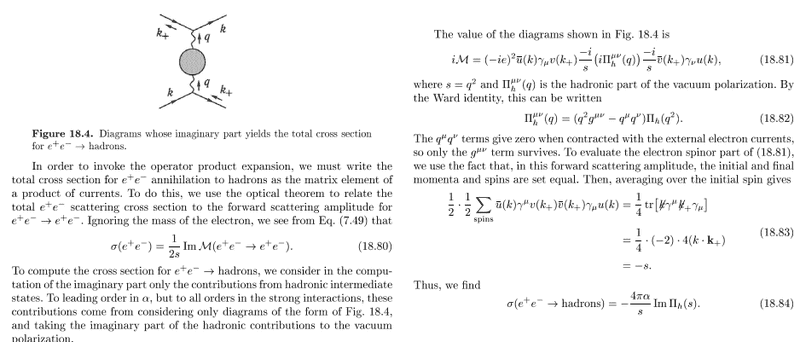# Peskin and Schroeder eq. 18.84

• qqbar

## Homework Statement

So I am self-studying the book of Peskin&Schroeder, and there is something I don't understand on page 616.

In eq. 18.80, there is a numerical factor of ½ and going from e2 to α will introduce a factor 4π when proceeding to eq. 18.84. But then there should be a numerical factor of 2π in eq 18.84. What gives an extra factor of 2?

## Homework Equations(this is the first time I post here, please do tell if uploading a page in this format is not acceptable)

## The Attempt at a Solution

I believe I understand the rest of the calculation on this page.

Last edited:
•DuckAmuck